Algebra is the part of math in which letters and symbols are used to represent numbers and quantities in equations. We’ve created an entirely new section of free worksheets for students to practice early algebra.

## Introduction to algebra vocabulary

We’ve created a series of algebra vocabulary worksheets to help students learn to differentiate between variables and constants, as well as expressions and equations.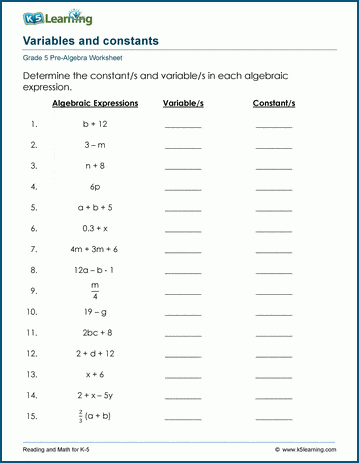## Practice expressions with one variable

These worksheets have students practice algebraic equations with one variable. They’ll need to know and understand the four operations and exponents before tackling these worksheets.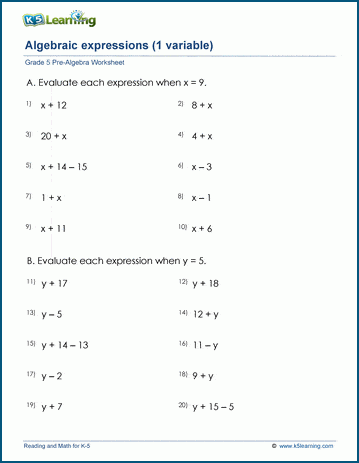## Practice expressions with two variables

These worksheets are more difficult than the previous section as they cover expressions with two variables.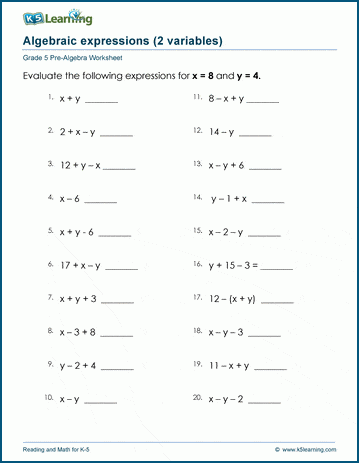## Writing algebraic expressions

Students are provided a short piece of text to write algebraic expressions in these worksheets.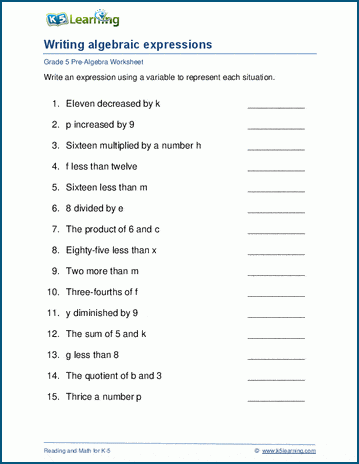## Learning to define variables

Students define variables and write expressions to answer questions about unknown amounts in these worksheets.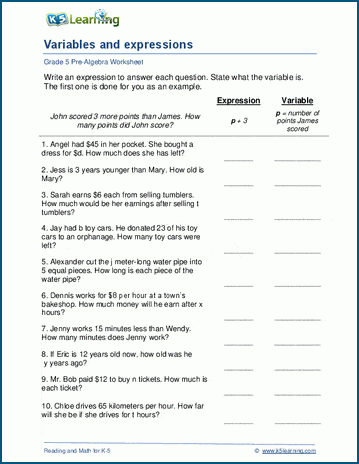## Worksheets to solve algebraic equations

These worksheets have students work on finding what x is. These worksheets are an introduction to learning to solve algebraic equations.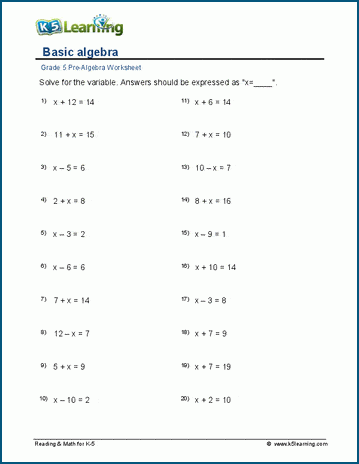## Learning to simplify expressions

Students combine like terms in these worksheets to simplify 1-variable expressions.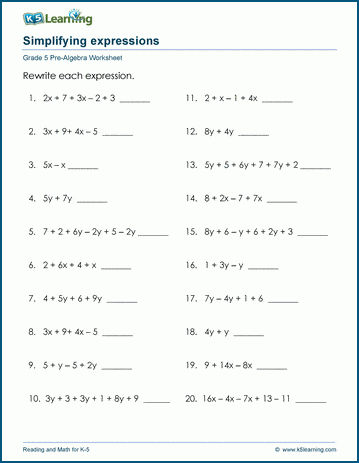## Practice algebra with fractions

For students looking to practice algebraic equations with fractions and decimals, these worksheets are a great start.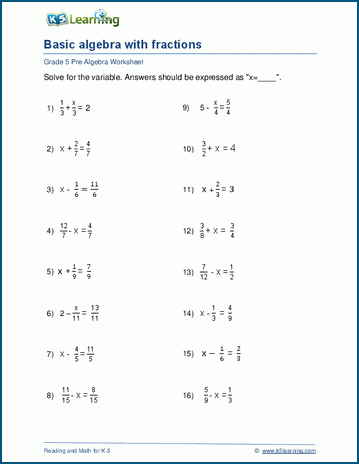## 2-step equations practice

Now things get a little harder. These algebra equations provide practice in simplifying and solving 1-variable equations.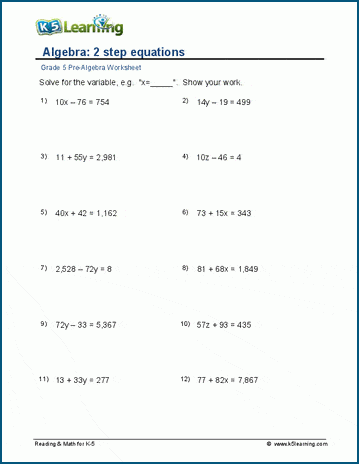## 2-sided equations practice

These are harder worksheets to practice simplifying and solving 1-variable equations. The answers will be solved in two steps.## Equations with 2 variables worksheets

Students work with tables to solve equations of x and y by evaluating expressions in the form y=f(x).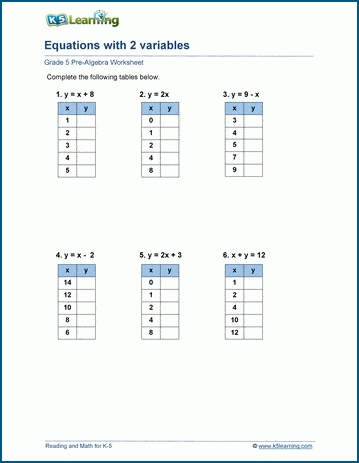Become a Member

This content is available to members only.